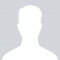Class Central is learner-supported. When you buy through links on our site, we may earn an affiliate commission.

## Overview

##### Class Central Tips
You've learned the basic algorithms now and are ready to step into the area of more complex problems and algorithms to solve them. Advanced algorithms build upon basic ones and use new ideas. We will start with networks flows which are used in more typical applications such as optimal matchings, finding disjoint paths and flight scheduling as well as more surprising ones like image segmentation in computer vision. We then proceed to linear programming with applications in optimizing budget allocation, portfolio optimization, finding the cheapest diet satisfying all requirements and many others. Next we discuss inherently hard problems for which no exact good solutions are known (and not likely to be found) and how to solve them in practice. We finish with a soft introduction to streaming algorithms that are heavily used in Big Data processing. Such algorithms are usually designed to be able to process huge datasets without being able even to store a dataset.

## Syllabus

• Flows in Networks
• Network flows show up in many real world situations in which a good needs to be transported across a network with limited capacity. You can see it when shipping goods across highways and routing packets across the internet. In this unit, we will discuss the mathematical underpinnings of network flows and some important flow algorithms. We will also give some surprising examples on seemingly unrelated problems that can be solved with our knowledge of network flows.
• Linear Programming
• Linear programming is a very powerful algorithmic tool. Essentially, a linear programming problem asks you to optimize a linear function of real variables constrained by some system of linear inequalities. This is an extremely versatile framework that immediately generalizes flow problems, but can also be used to discuss a wide variety of other problems from optimizing production procedures to finding the cheapest way to attain a healthy diet. Surprisingly, this very general framework admits efficient algorithms. In this unit, we will discuss some of the importance of linear programming problems along with some of the tools used to solve them.
• NP-complete Problems
• Although many of the algorithms you've learned so far are applied in practice a lot, it turns out that the world is dominated by real-world problems without a known provably efficient algorithm. Many of these problems can be reduced to one of the classical problems called NP-complete problems which either cannot be solved by a polynomial algorithm or solving any one of them would win you a million dollars (see Millenium Prize Problems) and eternal worldwide fame for solving the main problem of computer science called P vs NP. It's good to know this before trying to solve a problem before the tomorrow's deadline :) Although these problems are very unlikely to be solvable efficiently in the nearest future, people always come up with various workarounds. In this module you will study the classical NP-complete problems and the reductions between them. You will also practice solving large instances of some of these problems despite their hardness using very efficient specialized software based on tons of research in the area of NP-complete problems.
• Coping with NP-completeness
• After the previous module you might be sad: you've just went through 5 courses in Algorithms only to learn that they are not suitable for most real-world problems. However, don't give up yet! People are creative, and they need to solve these problems anyway, so in practice there are often ways to cope with an NP-complete problem at hand. We first show that some special cases on NP-complete problems can, in fact, be solved in polynomial time. We then consider exact algorithms that find a solution much faster than the brute force algorithm. We conclude with approximation algorithms that work in polynomial time and find a solution that is close to being optimal.
• Streaming Algorithms (Optional)
• In most previous lectures we were interested in designing algorithms with fast (e.g. small polynomial) runtime, and assumed that the algorithm has random access to its input, which is loaded into memory. In many modern applications in big data analysis, however, the input is so large that it cannot be stored in memory. Instead, the input is presented as a stream of updates, which the algorithm scans while maintaining a small summary of the stream seen so far. This is precisely the setting of the streaming model of computation, which we study in this lecture. The streaming model is well-suited for designing and reasoning about small space algorithms. It has received a lot of attention in the literature, and several powerful algorithmic primitives for computing basic stream statistics in this model have been designed, several of them impacting the practice of big data analysis. In this lecture we will see one such algorithm (CountSketch), a small space algorithm for finding the top k most frequent items in a data stream.

### Taught by

Alexander S. Kulikov and Michael Levin

## Reviews

3.0 rating, based on 1 reviews

•